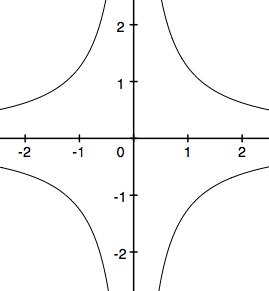High School Trigonometry: Help and Review Final Exam

Free Practice Test Instructions:

Choose your answer to the question and click 'Continue' to see how you did. Then click 'Next Question' to answer the next question. When you have completed the free practice test, click 'View Results' to see your results. Good luck!

Question 3 3. Which of the following shows the graph of the equation below?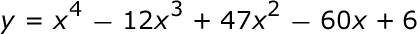Question 4 4. Simplify.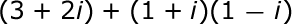Question 5 5. What is(are) the vertical asymptotes of the following function?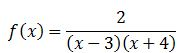Question 6 6. Which of the following is true?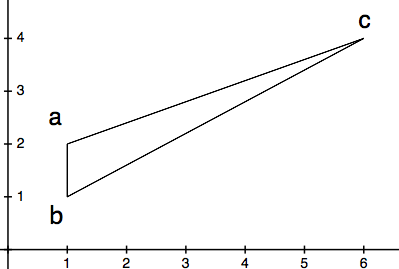Question 7 7. Convert the following measurement in radian to degrees.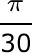Question 10 10. What type of solution(s) would you get from the equation below?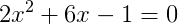Question 11 11. What is the function for the following graph?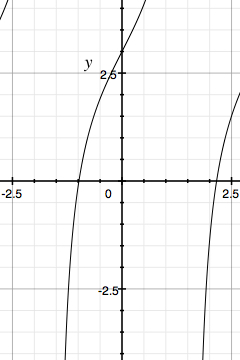Question 13 13. Subtract the following rational expressions.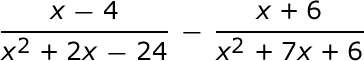Question 15 15. How many functions y=f(x) are needed to represent the graph below?